• 提出了一种利用卷积神经网络（CNN）和联合贝叶斯算法进行离线文本无关作者识别的新方法，该方法包括特征提取和作者识别两个阶段。在特征提取阶段，由于大量数据对于训练具有高泛化性的有效CNN模型是必不可少的，而且...
• 论文题目：Vehicle Re-Identification by Deep Feature Fusion Based on Joint Bayesian Criterion（ICPR） Abstract： 车辆重识别不同于行人重识别，其挑战性在于同种款式的不同车辆...通过提出的联合贝叶斯损...
论文题目：Vehicle Re-Identification by Deep Feature Fusion Based on Joint Bayesian Criterion（ICPR）

Abstract：

车辆重识别不同于行人重识别，其挑战性在于同种款式的不同车辆之间差异十分细小。本篇论文提出了双网络深度融合特征，两个网络可以提取输入图像的不同层面的特征并可以互相补充。通过提出的联合贝叶斯损失可以优化不同层面深度特征的融合，进而最小化类内间距，最大化类间间距，比较适合于车辆重识别任务。

Framework：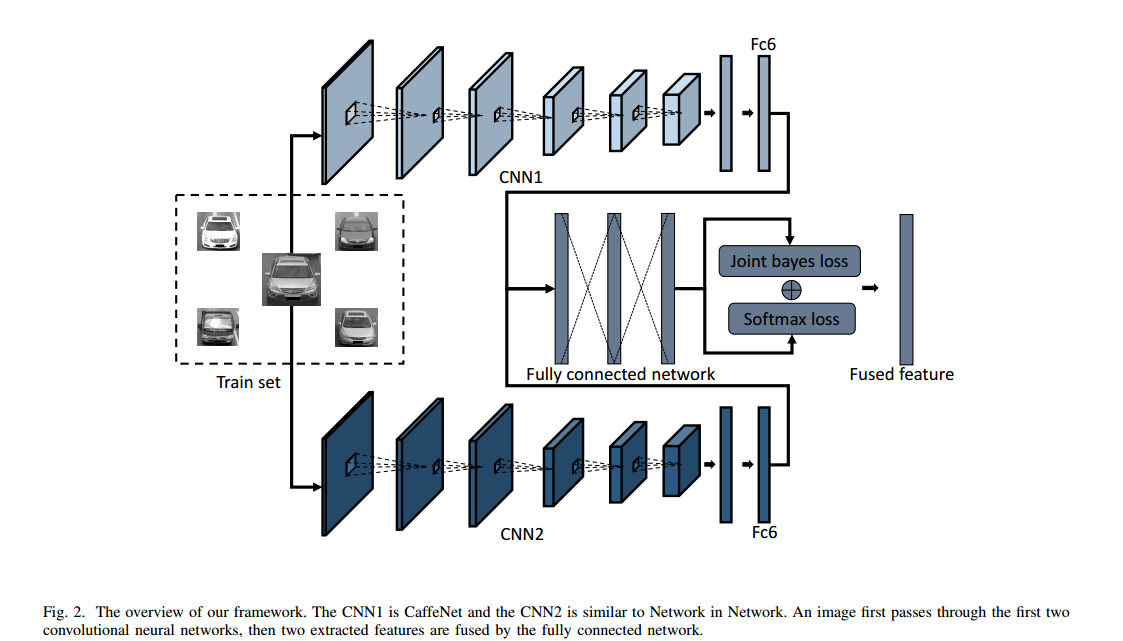本文使用两个CNN网络来提取不同层面的特征，CNN1为CaffeNet，CNN2类似于NIN，其MLP卷积层具有很好的局部抽象能力。在得到两个网络的特征后，使用三层全连接网络进行特征融合，最后使用Softmax损失与联合贝叶斯判别损失进行了联合优化，获取强大的判别能力。

CaffeNet是一个小型网络，主要由5个卷积层、3个池化层、2个norm层与3个全连接层。结构如下：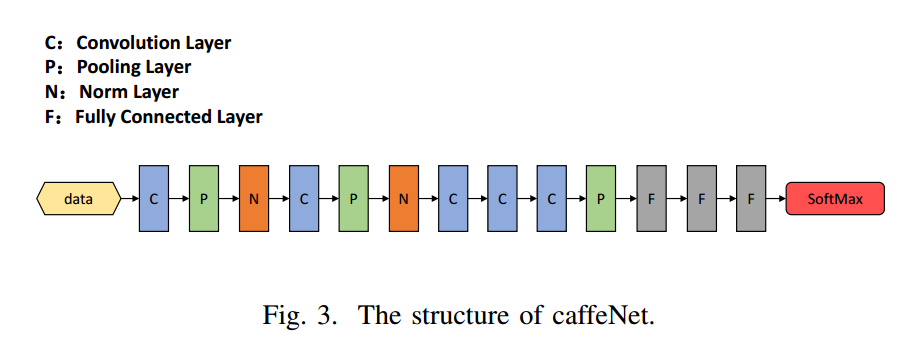NiN网络中的mlpconv卷积结构可以增强模型在感受野（receptive field）内对局部区域（local patches）的辨别能力。

类似的CNN2具体组成如下表：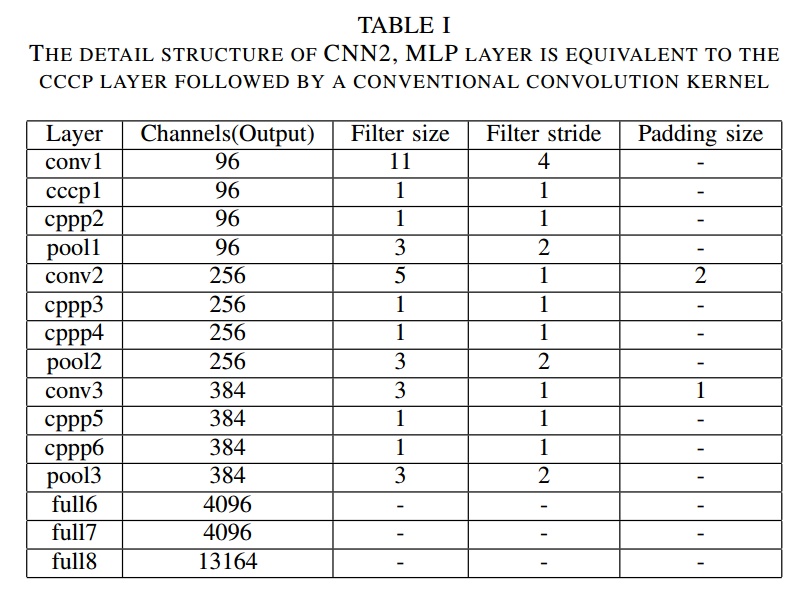普通卷积与mlp卷积的比较：

中间使用了两个1x1卷积与非线性操作，进行了所有通道间特征块的一个感知融合，具有更好的局部特征感知提取能力。贝叶斯判别损失：

贝叶斯方法在人脸验证应用中具有很好的效果。

车辆特征可以由两部分组成，一部分是正确的身份信息，一部分是身份偏差，两者都服从高斯分布。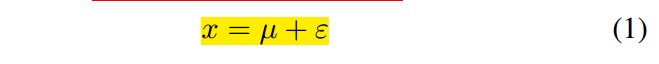其中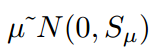，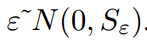，假设有两辆车的特征x1，x2，Hi表示同一车辆假设，He表示不同车辆假设。

两者的分布分别为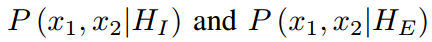，它们各自的协方差如下：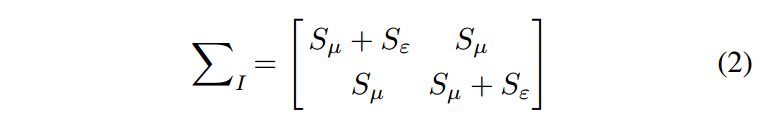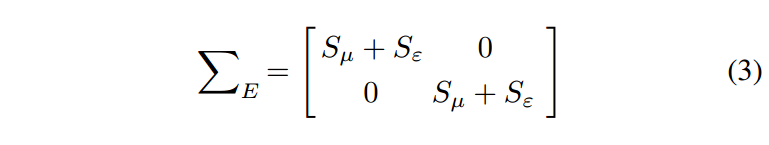联合贝叶斯概率比：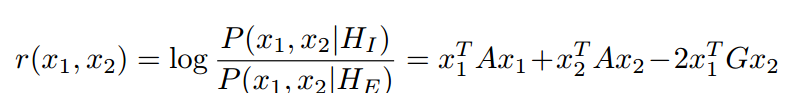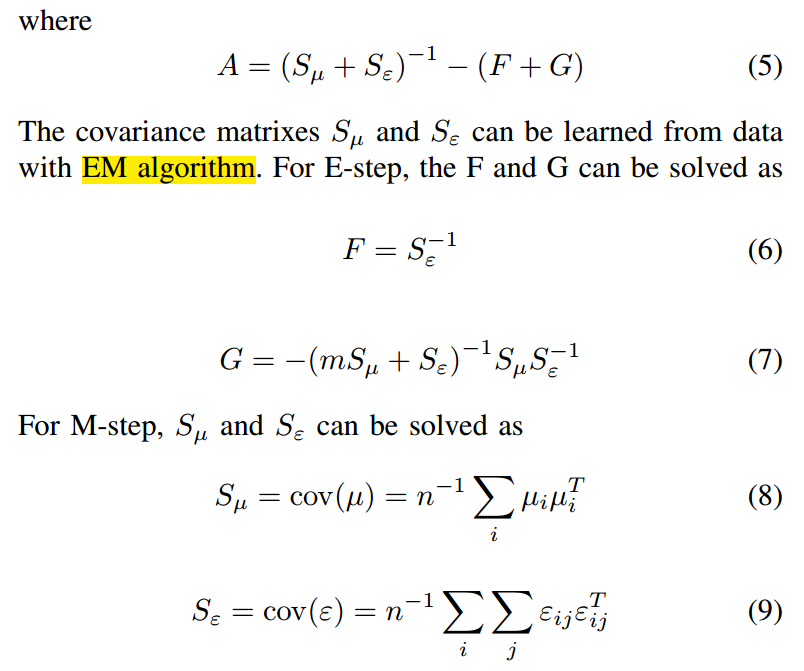贝叶斯损失如下定义：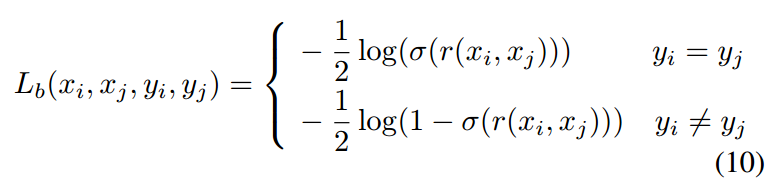softmax损失：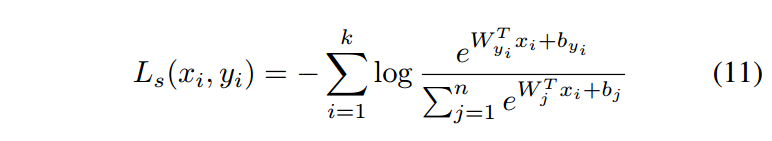网络的整体损失：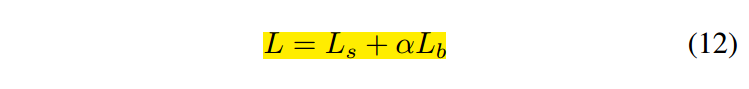实验结果：

试验在VehiclleID数据集上取得了很不错的效果，而且数据量越大，优势越明显。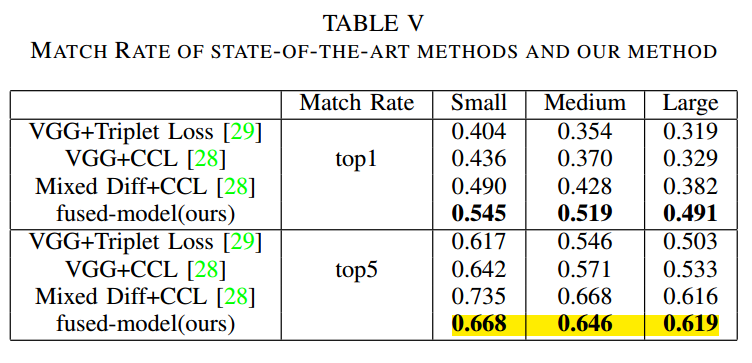总结：

多种层面特征融合是比较很好的应用技巧，而使用的贝叶斯损失很好地引导这个特征的融合。

展开全文• Joint Learning of J-Vector Extractor and Joint Bayesian Model for Text Dependent Speaker Verification ...用于文本相关说话人验证的J-Vector提取器和联合贝叶斯模型的联合学习 施自强，刘柳，林惠彬，刘如杰 ...
Joint Learning of J-Vector Extractor and Joint Bayesian Model for Text Dependent Speaker Verification

Ziqiang Shi, Liu Liu, Huibin Lin, Rujie Liu

用于文本相关说话人验证的J-Vector提取器和联合贝叶斯模型的联合学习
施自强，刘柳，林惠彬，刘如杰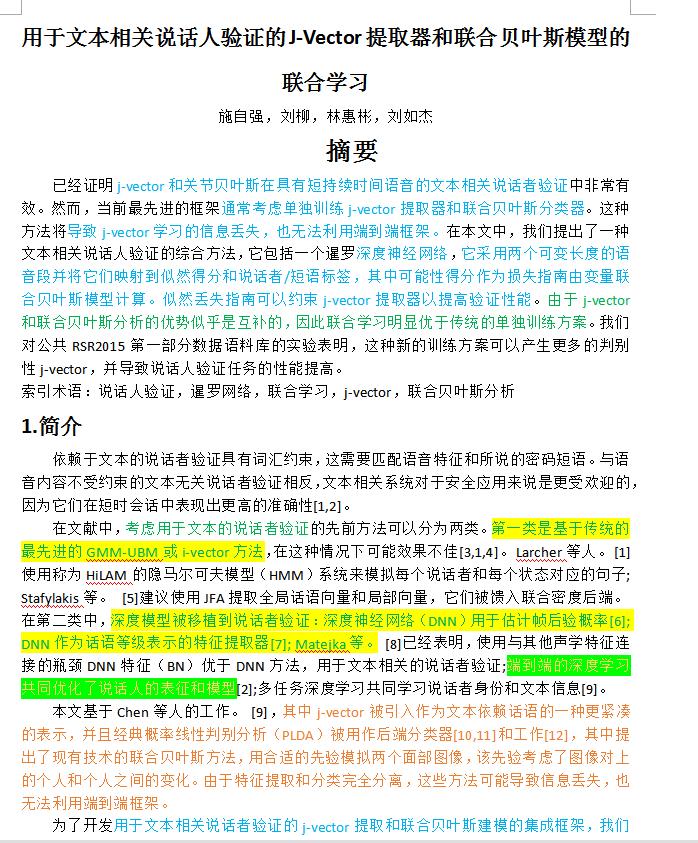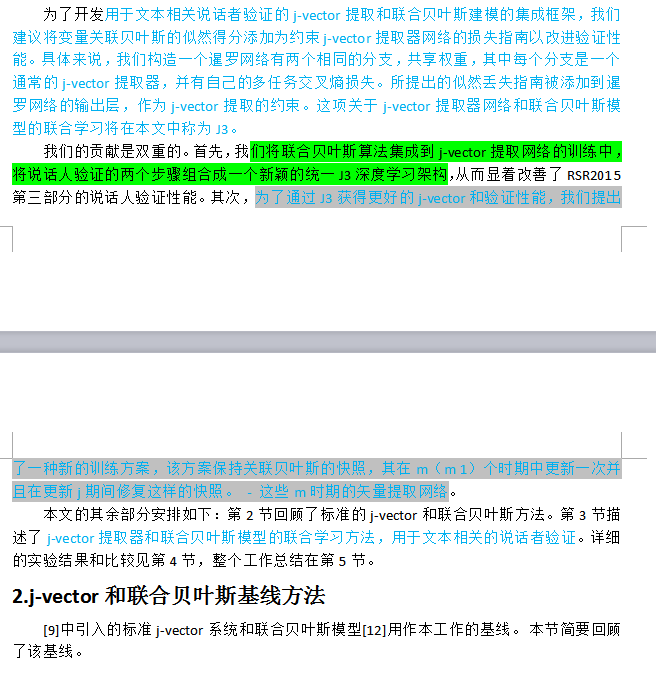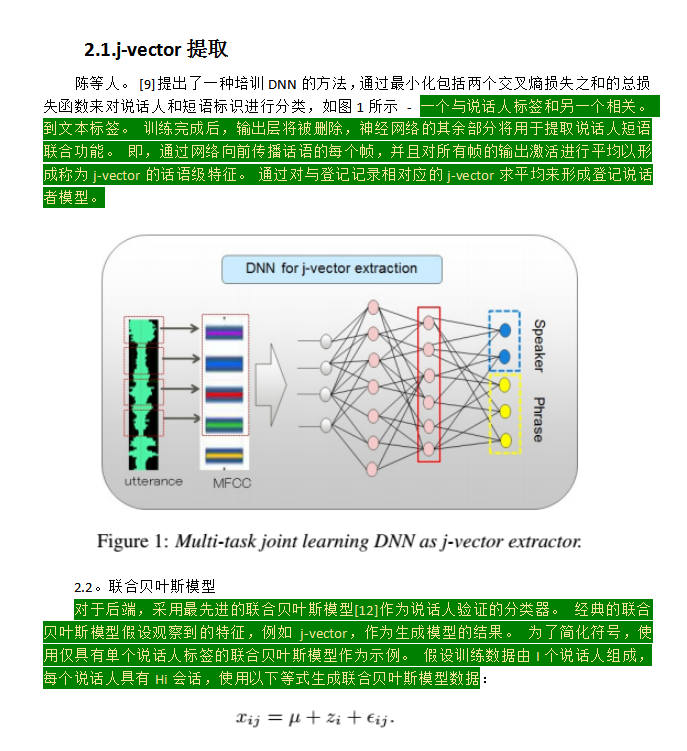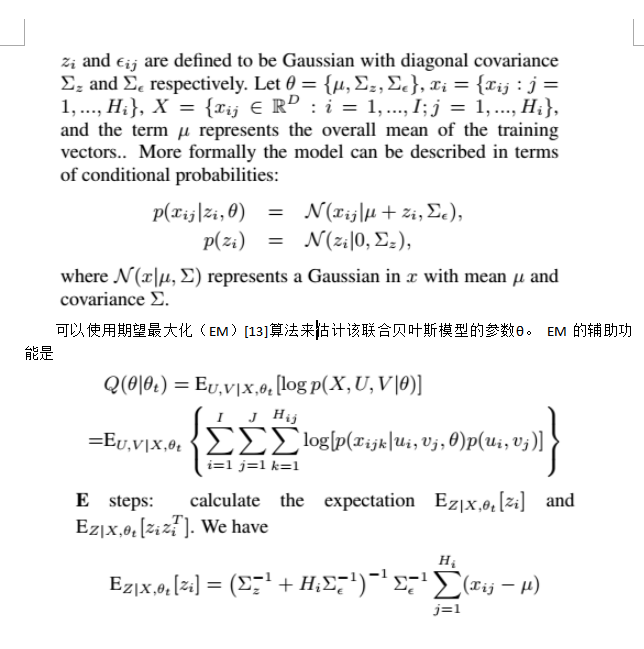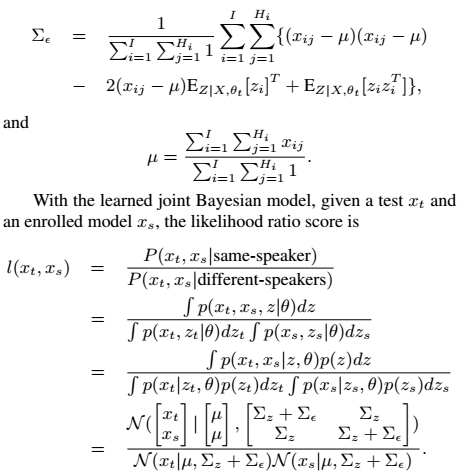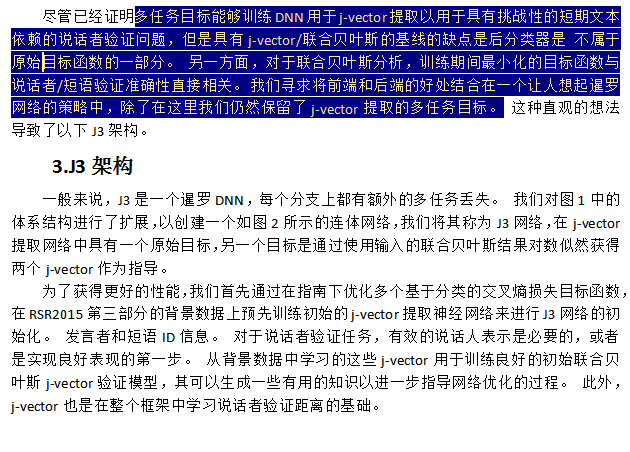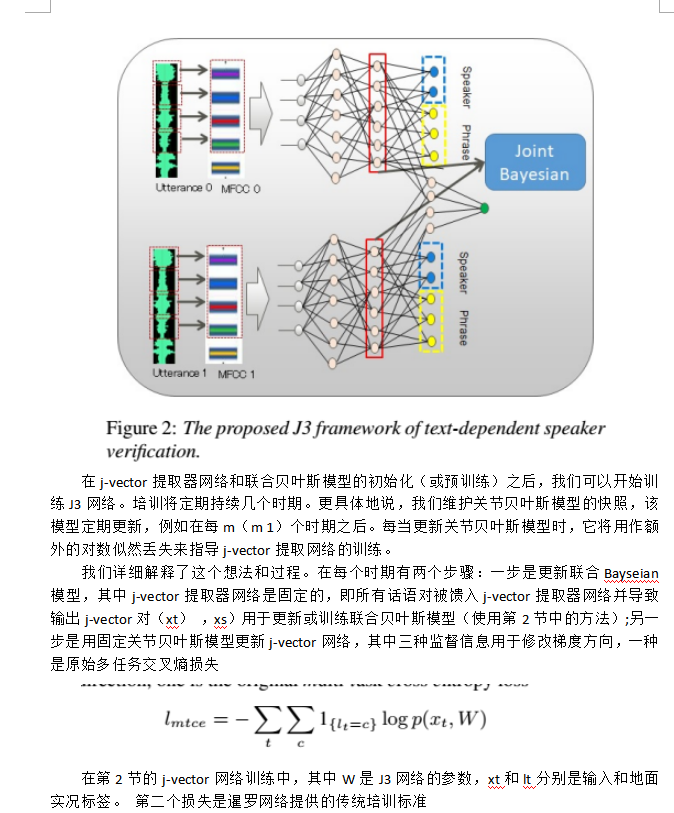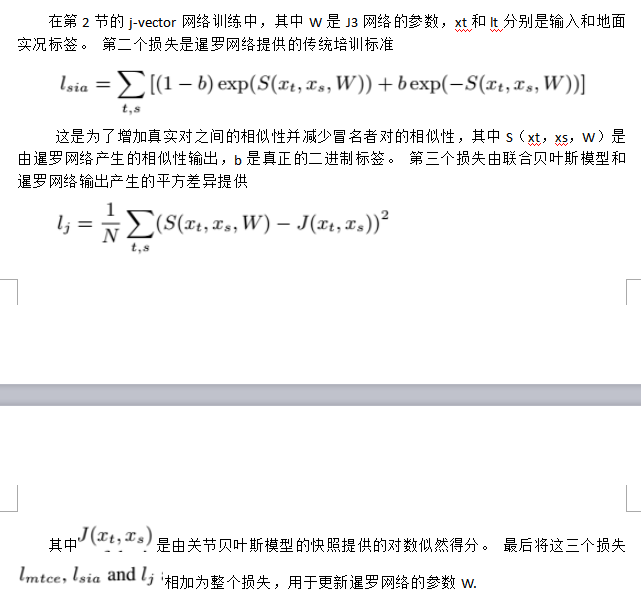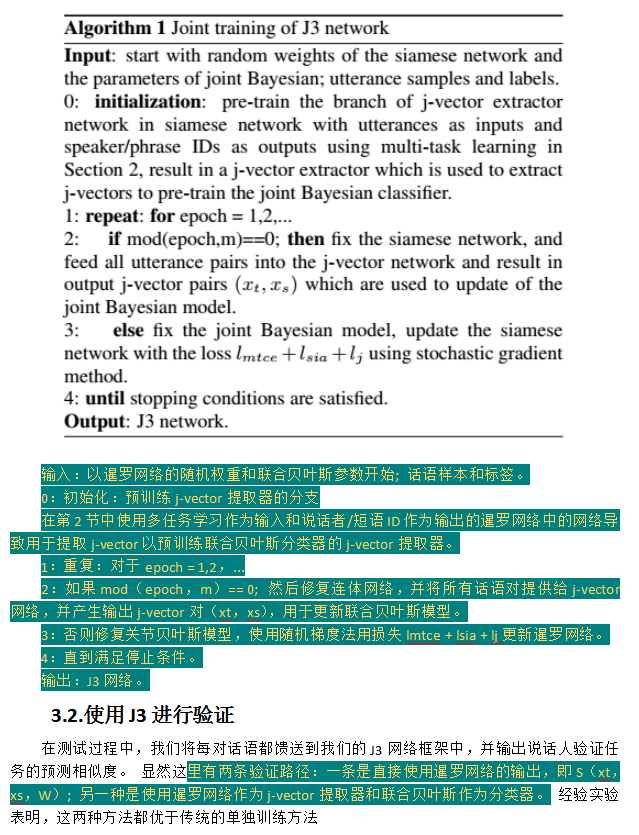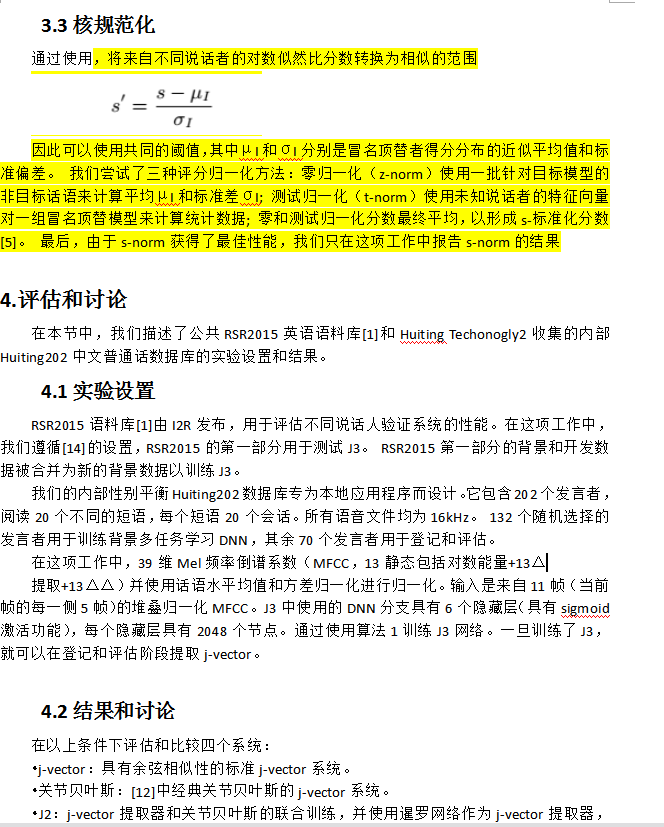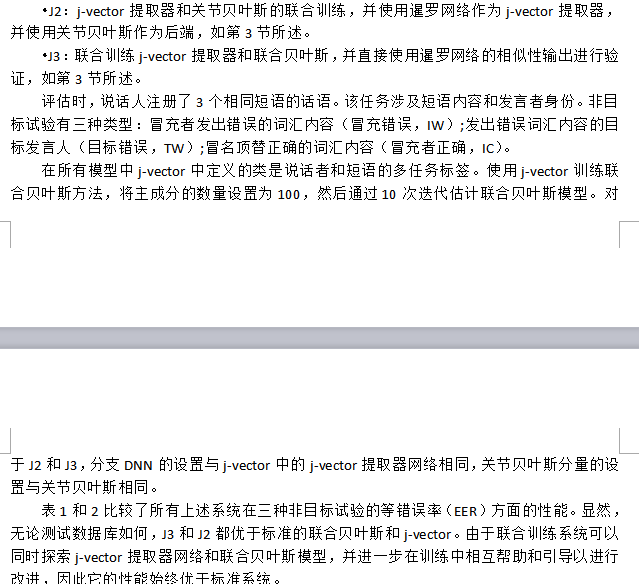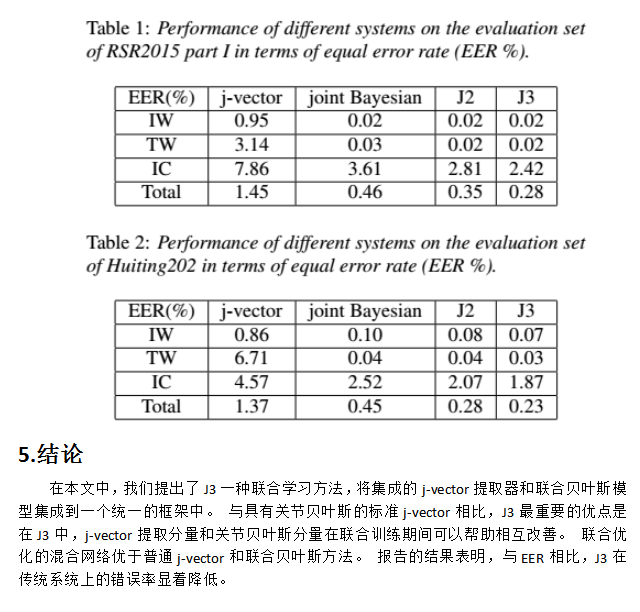展开全文• 1.特征表示 人脸由两个高斯变量的和表征：x=μ+εx=\mu+\varepsilonx=μ+ε 这里xxx代表人脸，μ\muμ代表固有身份，ε\varepsilonε代表脸部变化（光照，姿态，表情等）。隐变量μ\muμ代表固有身份，ε\varepsilon...
特征表示
人脸由两个高斯变量的和表征：$x=\mu+\varepsilon$
这里$x$代表人脸，$\mu$代表固有身份，$\varepsilon$代表脸部变化（光照，姿态，表情等）。隐变量$\mu$代表固有身份，$\varepsilon$服从高斯分布$\ N(0, \Sigma \varepsilon)$,$N\left(0, S_{\varepsilon}\right)$
以照片$x_{i}$和$x_{j}$为例,$x_{i}=\mu_{i}+\epsilon_{i}, x_{j}=\mu_{j}+\epsilon_{j}, i, j \in\{1,2\}$
\begin{aligned} \operatorname{cov}\left(x_{i}, x_{j}\right) &=\operatorname{cov}\left(\mu_{i}, \mu_{j}\right)+\operatorname{cov}\left(\mu_{i}, \epsilon_{j}\right)+\operatorname{cov}\left(\epsilon_{i}, \mu_{j}\right)+\operatorname{cov}\left(\epsilon_{i}, \epsilon_{j}\right) \\ &=\operatorname{cov}\left(\mu_{i}, \mu_{j}\right)+0+0+\operatorname{cov}\left(\epsilon_{i}, \epsilon_{j}\right) \quad(\text { Because } \epsilon \text { and } \mu \text { are independent }) \\ &=\operatorname{cov}\left(\mu_{i}, \mu_{j}\right)+\operatorname{cov}\left(\epsilon_{i}, \epsilon_{j}\right) \end{aligned}
判断依据推导
$p\left(x_{1}, x_{2} \mid H_{I}\right) \sim \mathcal{N}\left(0, \Sigma_{I}\right), \quad p\left(x_{1}, x_{2} \mid H_{E}\right) \sim \mathcal{N}\left(0, \Sigma_{E}\right)$
在HI假设下，即两张脸属于同一个人，本征变量μ1,μ2是相同的，而且ϵ1,ϵ2是相互独立的
$\Sigma_{I}=\left[\begin{array}{cc}S_{\mu}+S_{\epsilon} & S_{\mu} \\ S_{\mu} & S_{\mu}+S_{\epsilon}\end{array}\right]$
在HE假设下，μ,ϵ都是独立的
$\Sigma_{E}=\left[\begin{array}{cc}S_{\mu}+S_{\epsilon} & 0 \\ 0 & S_{\mu}+S_{\epsilon}\end{array}\right]$
$\Sigma_{I}^{-1}(仅仅作为标记)=\left(\begin{array}{cc} F+G & G \\ G & F+G \end{array}\right)$
$\Sigma_{E}^{-1}=\left(\begin{array}{cc} \left(S_{\mu}+S_{\epsilon}\right)^{-1} & 0 \\ 0 & \left(S_{\mu}+S_{\epsilon}\right)^{-1} \end{array}\right)$
$\Sigma_{E}$，$\Sigma_{I}$带入高斯公式可得：
$\begin{array}{l} p\left(x_{1}, x_{2} \mid H_{I}\right)=\frac{1}{(2 \pi)^{m / 2}\left|\Sigma_{I}\right|^{\frac{1}{2}}} \exp \left(-\frac{1}{2}\left(x_{1} \quad x_{2}\right) \Sigma_{I}^{-1}\left(\begin{array}{c} x_{1} \\ x_{2} \end{array}\right)\right) \\ p\left(x_{1}, x_{2} \mid H_{E}\right)=\frac{1}{(2 \pi)^{m / 2}\left|\Sigma_{E}\right|^{\frac{1}{2}}} \exp \left(-\frac{1}{2}\left(x_{1} \quad x_{2}\right) \Sigma_{E}^{-1}\left(\begin{array}{c} x_{1} \\ x_{2} \end{array}\right)\right) \end{array}$
可以得到：
$r\left(x_{1}, x_{2}\right)=\log \frac{p\left(x_{1}, x_{2} \mid H_{I}\right)}{p\left(x_{1}, x_{2} \mid H_{E}\right)}$
$=\log \frac{\left|\Sigma_{I}\right|^{-\frac{1}{2}} \exp \left(-\frac{1}{2}\left(x_{1} \quad x_{2}\right) \Sigma_{I}^{-1}\left(\begin{array}{l} x_{1} \\ x_{2} \end{array}\right)\right)}{\left|\Sigma_{E}\right|^{-\frac{1}{2}} \exp \left(-\frac{1}{2}\left(x_{1} \quad x_{2}\right) \Sigma_{E}^{-1}\left(\begin{array}{l} x_{1} \\ x_{2} \end{array}\right)\right)}$
\begin{aligned} &=\log \left[\exp \left(-\frac{1}{2}\left(x_{1} \quad x_{2}\right)\left(\begin{array}{c} \left.\Sigma_{E}^{-1}-\Sigma_{I}^{-1}\right) \\ x_{2} \end{array}\right)\right) \cdot \frac{\left|\Sigma_{I}\right|^{-\frac{1}{2}}}{\left|\Sigma_{E}\right|^{-\frac{1}{2}}}\right.\\ &=-\frac{1}{2}\left(\begin{array}{ll} x_{1} & x_{2} \end{array}\right)\left(\begin{array}{l} \left.\Sigma_{E}^{-1}-\Sigma_{I}^{-1}\right) \\ x_{2} \end{array}\right)+C_{1} \quad\left(\text { here } C_{1}=\log \frac{\left|\Sigma_{I}\right|^{-\frac{1}{2}}}{\left|\Sigma_{E}\right|^{-\frac{1}{2}}}\right)\\ &=\frac{1}{2}\left(\begin{array}{ll} x_{1} & x_{2} \end{array}\right)\left(\left(\begin{array}{cc} \left(S_{\mu}+S_{\epsilon}\right)^{-1} & 0 \\ 0 & \left(S_{\mu}+S_{\epsilon}\right)^{-1} \end{array}\right)-\left(\begin{array}{cc} F+G & G \\ G & F+G \end{array}\right)\right)\left(\begin{array}{l} x_{1} \\ x_{2} \end{array}\right)+C_{1}\\ &=\frac{1}{2}\left(\begin{array}{ll} x_{1} & x_{2} \end{array}\right)^{T}\left(\begin{array}{cc} A & -G \\ -G & A \end{array}\right)\left(\begin{array}{ll} x_{1} & x_{2} \end{array}\right)+C_{1}\\ &=\frac{1}{2}\left(x_{1}^{T} A x_{1}-2 x_{1}^{T} G x_{2}+x_{2}^{T} x_{2}\right)+C_{1} \end{aligned}
可得$A=\left(S_{\mu}+S_{\epsilon}\right)^{-1}-(F+G)$
推导$\Sigma_{x}$
$x_{1}=\mu+\epsilon_{1}, \quad x_{2}=\mu+\epsilon_{2}, \quad \ldots, \quad x_{m}=\mu+\epsilon_{m}$
$x=\left(\begin{array}{c} x_{1} \\ x_{2} \\ \vdots \\ x_{m} \end{array}\right)_{m * 1}, \quad P=\left(\begin{array}{ccccc} 1 & 1 & 0 & \dots & 0 \\ 1 & 0 & 1 & \dots & 0 \\ \vdots & \vdots & \vdots & \ddots & \vdots \\ 1 & 0 & 0 & \dots & 1 \end{array}\right)_{(m) *(m+1)}, \quad h=\left(\begin{array}{c} \mu_{1} \\ \epsilon_{2} \\ \vdots \\ \epsilon_{m} \end{array}\right)_{(m+1) * 1}$
$x=P h$
$h \sim \mathcal{N}\left(0, \Sigma_{h}\right), \quad \Sigma_{h}=\left(\begin{array}{cccc} S_{\mu} & 0 & \cdots & 0 \\ 0 & S_{\epsilon} & \cdots & 0 \\ \vdots & \vdots & \ddots & \vdots \\ 0 & 0 & \cdots & S_{\epsilon} \end{array}\right)$
依据$\operatorname{Cov}[\mathbf{A} \mathbf{x}, \mathbf{B y}]=\mathbf{A} \operatorname{Cov}[\mathbf{x}, \mathbf{y}] \mathbf{B}^{T}$
$x \sim \mathcal{N}\left(0, \Sigma_{x}\right), \quad \Sigma_{x}=P \Sigma_{h} P^{T}=\left(\begin{array}{cccc} S_{\mu}+S_{\epsilon} & S_{\mu} & \dots & S_{\mu} \\ S_{\mu} & S_{\mu}+S_{\epsilon} & \dots & S_{\mu} \\ \vdots & \vdots & \ddots & \vdots \\ S_{\mu} & S_{\mu} & \dots & S_{\mu}+S_{\epsilon} \end{array}\right)$
关于推导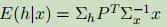依据：
$\begin{array}{l} \text { Assume } \mathbf{x} \sim \mathcal{N}_{\mathbf{x}}(\mu, \mathbf{\Sigma}) \text { where } \\ \qquad \mathbf{x}=\left[\begin{array}{c} \mathbf{x}_{a} \\ \mathbf{x}_{b} \end{array}\right] \quad \boldsymbol{\mu}=\left[\begin{array}{c} \boldsymbol{\mu}_{a} \\ \boldsymbol{\mu}_{b} \end{array}\right] \quad \mathbf{\Sigma}=\left[\begin{array}{cc} \boldsymbol{\Sigma}_{a} & \boldsymbol{\Sigma}_{c} \\ \boldsymbol{\Sigma}_{c}^{T} & \boldsymbol{\Sigma}_{b} \end{array}\right] \end{array}$
$p\left(\mathbf{x}_{a} \mid \mathbf{x}_{b}\right)=\mathcal{N}_{\mathbf{x}_{a}}\left(\hat{\mu}_{a}, \hat{\mathbf{\Sigma}}_{a}\right) \quad\left\{\begin{array}{l} \hat{\boldsymbol{\mu}}_{a}=\boldsymbol{\mu}_{a}+\boldsymbol{\Sigma}_{c} \mathbf{\Sigma}_{b}^{-1}\left(\mathbf{x}_{b}-\boldsymbol{\mu}_{b}\right) \\ \hat{\mathbf{\Sigma}}_{a}=\boldsymbol{\Sigma}_{a}-\boldsymbol{\Sigma}_{c} \mathbf{\Sigma}_{b}^{-1} \boldsymbol{\Sigma}_{c}^{T} \end{array}\right.$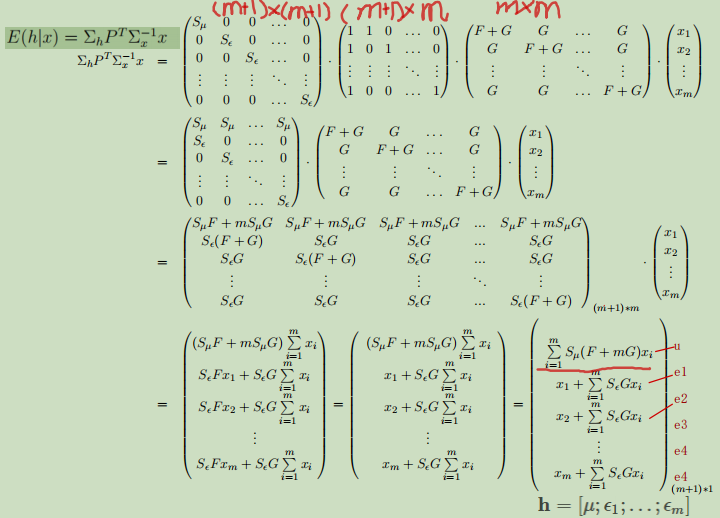因此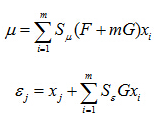求解F,G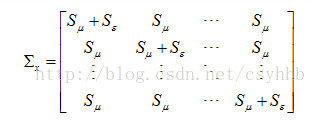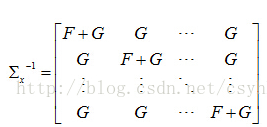根据相乘玩对角线为一，非对角线为零可得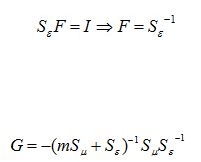流程图：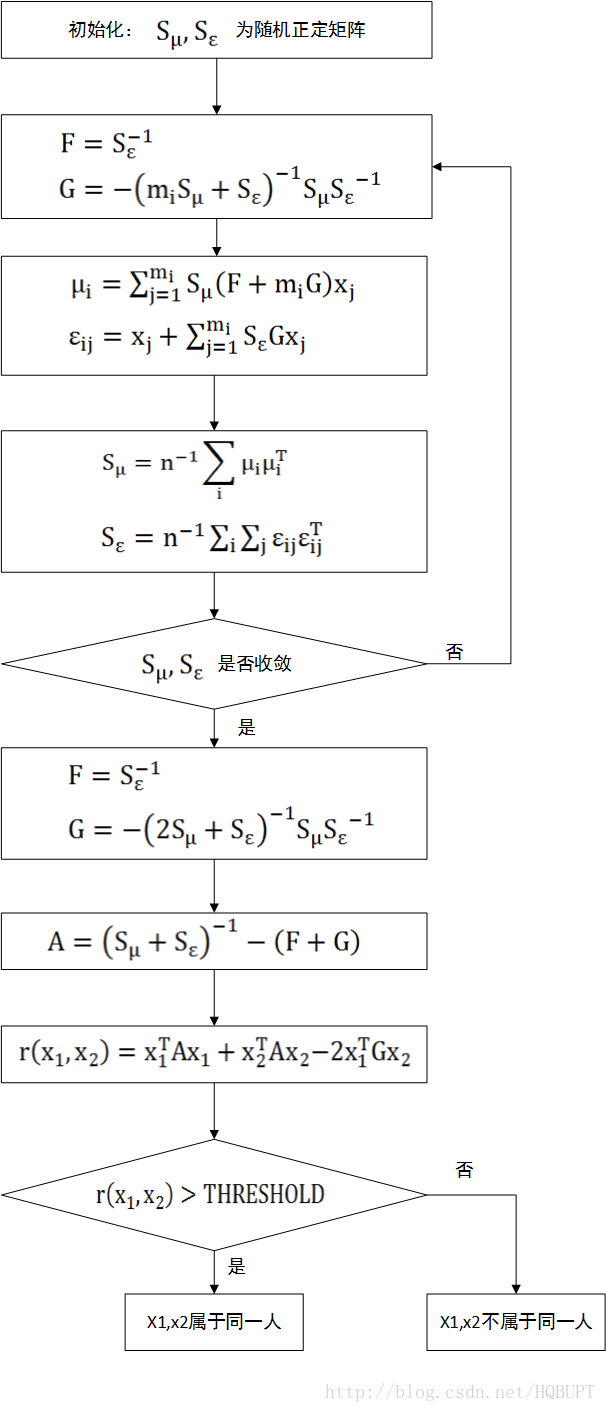1.初始化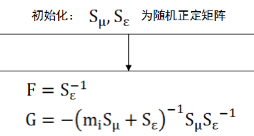2，EMlike算法
E步：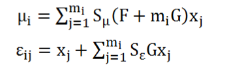M步：更新参数{Sμ,Sϵ}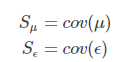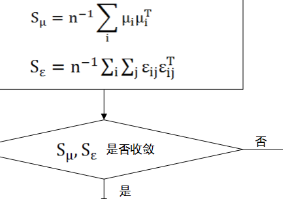3.联合贝叶斯判据


展开全文• 特征分析在计算机视觉和计算机...本文提出了一种有效且鲁棒的三维模型描述符，基于表面拉普拉斯-贝尔特拉米算子的归一化特征函数的概率分布和用于降维的频谱方法来构造描述符，利用联合贝叶斯模型来估计描述符空间中...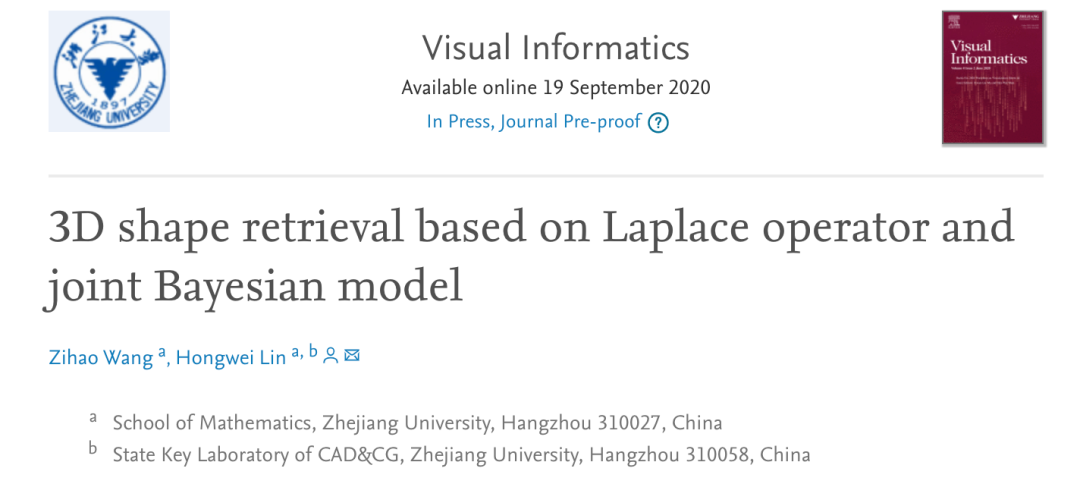特征分析在计算机视觉和计算机图形学中起着重要的作用。在形状检索的任务中，形状描述符必不可少。近年来，基于深度学习的特征提取非常流行，但是由于各种形状所包含的内在信息及其可解释性，对它们的几何形状描述符的设计仍然很有意义。本文提出了一种有效且鲁棒的三维模型描述符，基于表面拉普拉斯-贝尔特拉米算子的归一化特征函数的概率分布和用于降维的频谱方法来构造描述符，利用联合贝叶斯模型来估计描述符空间中的距离，在训练阶段引入矩阵正则化过程来重新估计协方差矩阵。最后，将3D形状检索描述符应用公共基准库。实验表明，该方法鲁棒，具有良好的检索性能。关键词：拉普拉斯-贝尔特拉米算子；联合贝叶斯模型；形状检索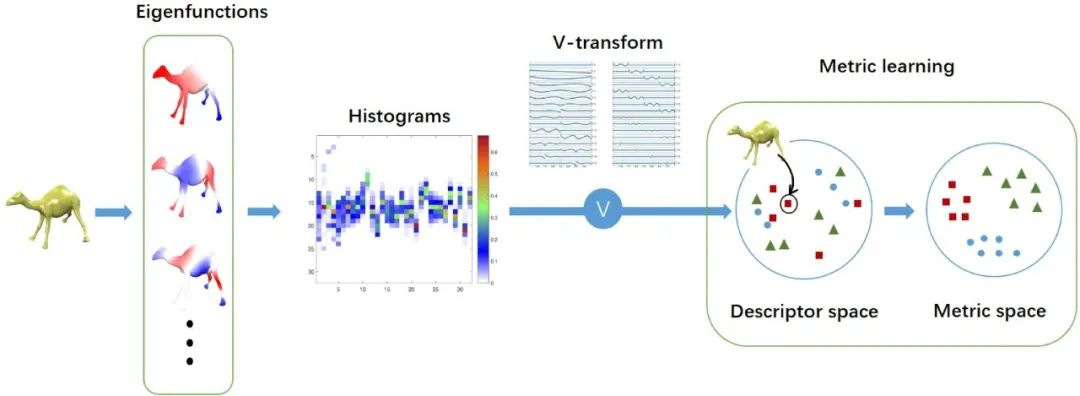Fig.1.  The pipeline of our approach.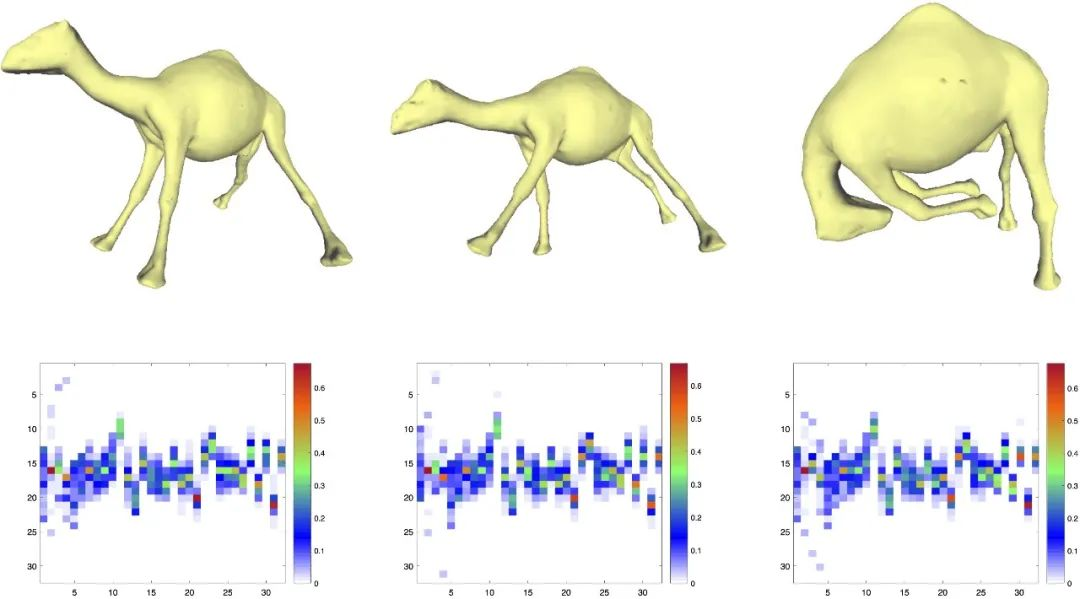Fig.2. Visualization of the IHs of size 32 × 32. The three 3D models undergone isometric transformation.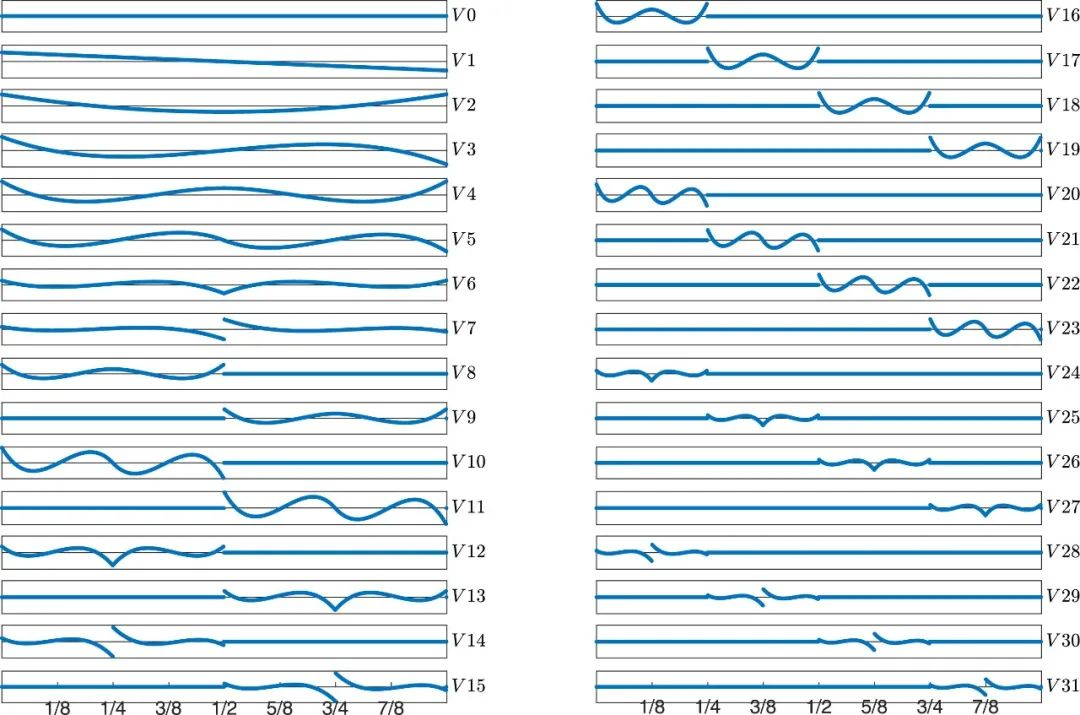Fig.3.  The first 32 basis functions in the V-system of degree 3. confidence intervals.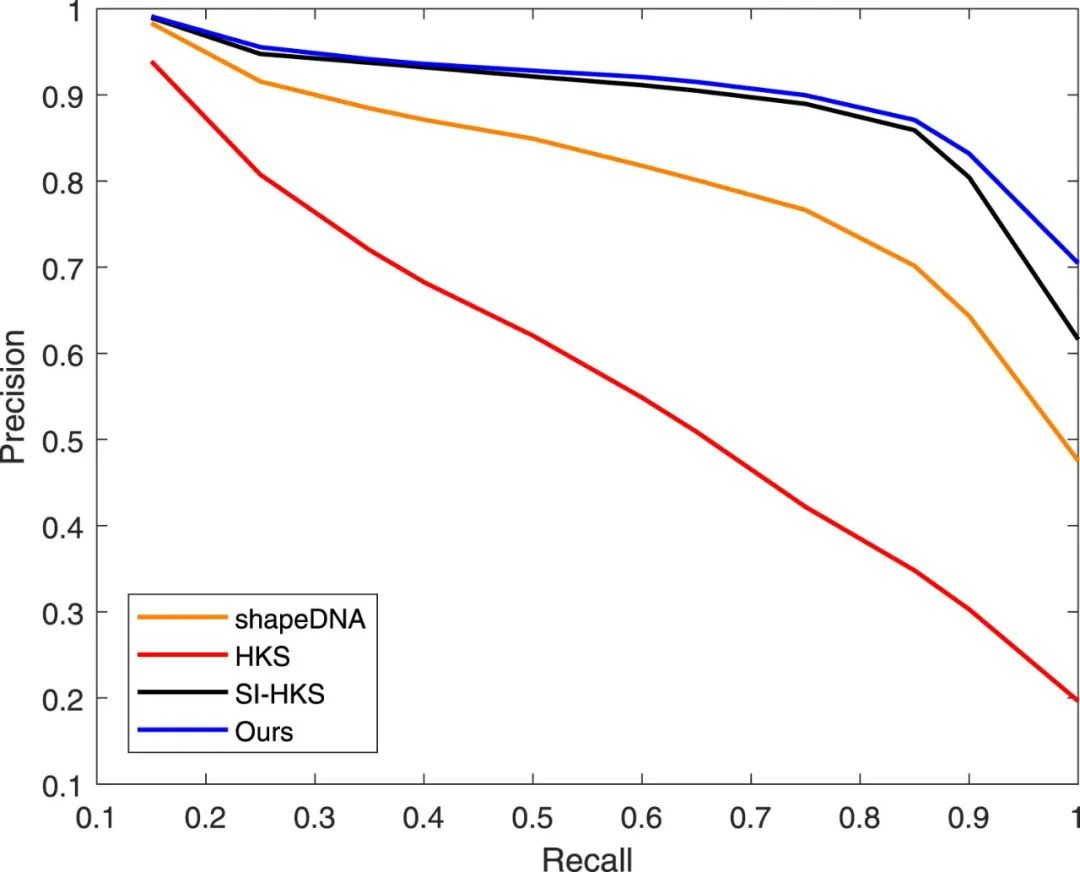Fig. 4. Retrieval performance of different descriptor under the framework in this paper.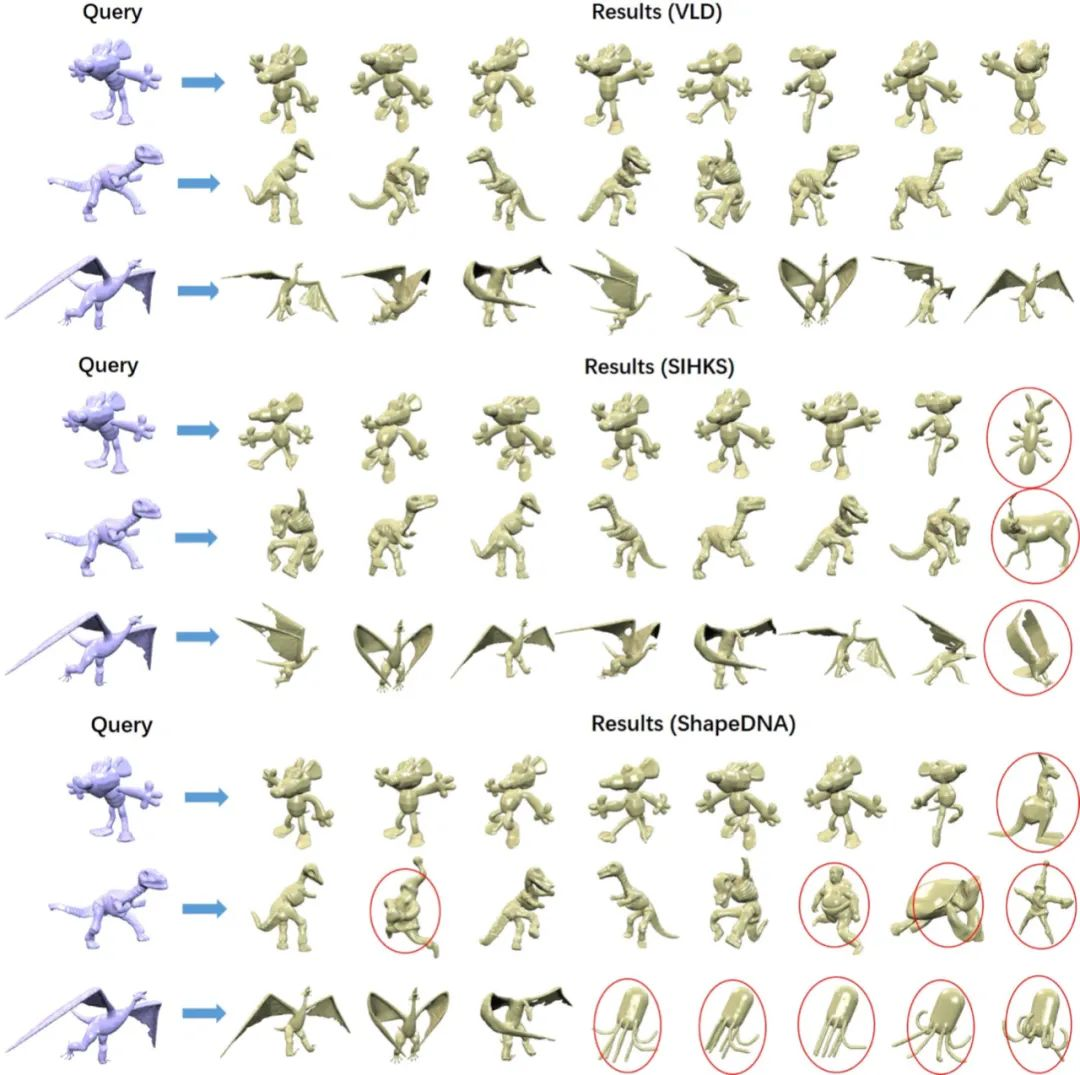Fig. 5. Results of non-rigid shape retrieval by using VLD, SIHKS and shapeDNA respectively. Each row represents the retrieval result using the 3D model in blue as the query model. Top 8 retrieval results are listed in the figure, and incorrect results are marked with red circles.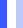HighlightsA shape descriptor is developed based on the V-transformation of the Laplace-Beltrami eigenfunctions.The metric of the descriptor space is learned by the joint Bayesian method and matrix regularization.Experiments show the robustness and effectiveness of the developed descriptor and the learned metric.全文信息3D shape retrieval based on Laplace operator and joint Bayesian modelBY:  Zihao Wang，Hongwei LinAbstract: Feature analysis plays a significant role in computer vision and computer graphics. In the task of shape retrieval, shape descriptor is indispensable. In recent years, feature extraction based on deep learning becomes very popular, but the design of geometric shape descriptor is still meaningful due to the contained intrinsic information and interpretability. This paper proposes an effective and robust descriptor of 3D models. The descriptor is constructed based on the probability distribution of the normalized eigenfunctions of the Laplace-Beltrami operator on the surface, and a spectrum method for dimensionality reduction. The distance metric of the descriptor space is learned by utilizing the joint Bayesian model, and we introduce a matrix regularization in the training stage to re-estimate the covariance matrix. Finally, we apply the descriptor to 3D shape retrieval on a public benchmark. Experiments show that our method is robust and has good retrieval performance.Keywords: Laplace-Beltrami operator，Joint Bayesian，Shape retrievalLink: https://doi.org/10.1016/j.visinf.2020.08.002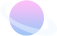END推荐阅读1、不同任务背景下对配有文本修饰的叙事式可视化的凝视行为分析2、自动信息图和可视化推荐综述3、DataV: 面向大尺度高分辨率显示装置的敏捷可视化设计与实现4、影响因子公布，计算机科学类看这里！5、TVseer：一个电视收视率的可视分析系统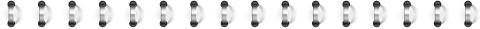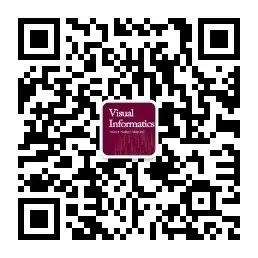Visual Informatics可视信息学An Open Access Journal网址：elsevier.com/locate/visinf邮箱：visinf@cad.zju.edu.cn长按扫码可关注点击此处“阅读全文”查看下载原文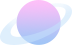展开全文• 在下菜鸟一枚，正在学习人脸识别相关内容，参考一位牛人的python版实现了C++版，并用C++/CLI封装，可供C#程序调用。python版实现：http://blog.csdn.net/cyh_24/article/details/49059475。  程序中使用了matlab...人脸识别 C++ C++CLI
• <span xss=removed><span> </span></span>将稀疏贝叶斯学习引入线性混合像元分解中，提出一种基于复合正则化联合稀疏贝叶斯学习的高光谱稀疏解混算法。在多观测向量的稀疏贝叶斯框架下，对各参数建立概率模型，经...
•研究论文
•研究论文
• 贝叶斯分类是一类以贝叶斯定理为基础的分类算法的总称，其中基于特征之间相互独立的朴素贝叶斯是最简单，但效果却非常好的一种分类算法。本文整理了贝叶斯算法的基本原理，希望能够对大家有所帮助~~ 一、贝叶斯定理 ...拉普拉斯平滑
• 1. 什么是朴素贝叶斯？ 朴素贝叶斯是一种直接...联合概率 “X取值为x”和“Y取值为y”两个事件同时发生的概率，即P(X=x,Y=y) 条件概率 在 “X取值为x”的前提下，“Y取值为y”的概率，即P(Y=y|X=x) 举个栗子来说明一下
• 贝叶斯法则通常，事件A在事件B(发生)的条件下的概率，与事件B在事件A的条件下的概率是不一样的；然而，这两者是有确定的关系,贝叶斯法则就是这种关系的陈述。作为一个规范的原理，贝叶斯法则对于所有概率的解释是...
• 有关评估贝叶斯网络的相关算法以及证明过程，本文比较了以往的一些传统算法与提出的联合概率分布评估贝叶斯网络做出了比较。
•研究论文
•研究论文
•研究论文
• 条件概率，边缘概率，联合概率，全概率，贝叶斯定理是机器学习中几乎所有算法的基础，虽然公式记住了，但是为什么是这样，以及如何理解看过之后隔一段时间问题忘，这里在这里好好做下总结 边缘概率 边缘概率是与联合...条件概率
• 四、如何把联合概率公式、条件概率公式变成贝叶斯公式 A和B一同发生的概率的联合概率： P(B,A) = f(A∩B)/f(O) = f(A∩B)/f(A) * f(A)/f(O) =P(B|A) * P(A) 注：可以理解成A和B一同发生的概率，就是A发生的概率，...算法
• 基于多祯数据联合处理的机载单通道雷达贝叶斯前视成像研究论文
• 基于广义贝叶斯风险的递归联合轨迹间关联和传感器非线性偏差估计研究论文
• 基于稀疏贝叶斯学习的OFDM系统联合信道估计和脉冲噪声抑制方法。研究论文
• （如需交流，请关注公众号：神马观止） Viusal C++6.0与Hugin Lite8.0联合编程构建贝叶斯网络推理机
• 其中的核心思想是利用变量之间的“朴素”性质，计算出联合概率密度。这依赖于朴素贝叶斯分类的一个限制条件，就是特征属性必须有条件独立或基本独立。但现实中各个特征属性间往往并不条件独立，而是具有较强的相关性...
• 联合概率和条件概率 相互独立的意思是例如，职业和体重两个条件是没有关系的，如果有关系的特征不能用，比如不锻炼和体重的关系 朴素贝叶斯（特征独立） 朴素贝叶斯会求其属于每个目标类别的概率，然后...
• 1、应用场景 1.1 垃圾邮件分类 2、概率基础 概率定义为一件事情发生的可能性 问题： 1、女神喜欢的概率？...2.1 联合概率和条件概率 以下主要来自：https://blog.csdn.net/sinat_30353259/article/deta...
• class bayes: def __init__(self,A,B,i,j): self.A = A self.B = B self.i = i self.j = j def joint_probability(self):... PAB = self.A*self.B #联合概率 return PAB def contingent_probability(self):
• 基于特征条件独立假设学习 输入/输出 联合分布 基于此模型 对于给定输入x，利用贝叶斯原理求出后验概率最大的输出y 基本方法： 贝叶斯估计优缺点 优点： 逻辑简单，容易实现 分类时空开销少 缺点 朴素...
• 基于块稀疏贝叶斯学习的无授权NOMA系统联合用户活动检测和信道估计研究论文
• 朴素贝叶斯算法基本原理 朴素贝叶斯法利用贝叶斯定理首先求出联合概率分布，再求出条件概率分布。这里的朴素是指在计算似然估计时假定了条件独立。基本原理可以用下面的公式给出： 其中， P(Y|X)叫做后验概率，P(Y)......